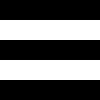PHP 8.0.24 Released!

# Imagick::importImagePixels

(PECL imagick 2 >= 2.3.0, PECL imagick 3)

Imagick::importImagePixelsImports image pixels

### Description

public Imagick::importImagePixels(
int `\$x`,
int `\$y`,
int `\$width`,
int `\$height`,
string `\$map`,
int `\$storage`,
array `\$pixels`
): bool

Imports pixels from an array into an image. The `map` is usually 'RGB'. This method imposes the following constraints for the parameters: amount of pixels in the array must match `width` x `height` x length of the map. This method is available if Imagick has been compiled against ImageMagick version 6.4.5 or newer.

### Parameters

`x`

The image x position

`y`

The image y position

`width`

The image width

`height`

The image height

`map`

Map of pixel ordering as a string. This can be for example `RGB`. The value can be any combination or order of R = red, G = green, B = blue, A = alpha (0 is transparent), O = opacity (0 is opaque), C = cyan, Y = yellow, M = magenta, K = black, I = intensity (for grayscale), P = pad.

`storage`

The pixel storage method. Refer to this list of pixel constants.

`pixels`

The array of pixels

### Return Values

Returns `true` on success.

### Errors/Exceptions

Throws ImagickException on error.

### Examples

Example #1 Imagick::importImagePixels() example

``` <?php/* Generate array of pixels. 2000 pixels per color stripe */\$count = 2000 * 3;\$pixels =    array_merge(array_pad(array(), \$count, 0),               array_pad(array(), \$count, 255),                array_pad(array(), \$count, 0),               array_pad(array(), \$count, 255),               array_pad(array(), \$count, 0));/* Width and height. The area is amount of pixels divided   by three. Three comes from 'RGB', three values per pixel */\$width = \$height = pow((count(\$pixels) / 3), 0.5);/* Create empty image */\$im = new Imagick();\$im->newImage(\$width, \$height, 'gray');/* Import the pixels into image.   width * height * strlen("RGB") must match count(\$pixels) */\$im->importImagePixels(0, 0, \$width, \$height, "RGB", Imagick::PIXEL_CHAR, \$pixels);/* output as jpeg image */\$im->setImageFormat('jpg');header("Content-Type: image/jpg");echo \$im;?> ```

The above example will output something similar to: